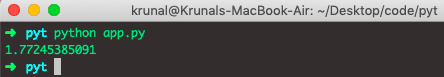# Python sqrt: How To Calculate Square Root in Python

In algebra, the square results from a number, n, multiplied by itself, which means n. So the equation becomes x = n². To calculate the square of a number in Python, check out the square tutorial.

The square root of the number is a number n, which, when multiplied by itself, yields the square, x. Let’s see how to calculate the square root of a number in the Python programming language.

## How to Calculate Square root in Python

To calculate a square root of the value in Python, use the sqrt() method. The sqrt() is a built-in Python math library function that returns the square root of x for x > 0. To use the sqrt() method, import the math module.

For example, we can import the math module and then call the sqrt() function to get the square root of any given value.

### Syntax

The syntax for the Python sqrt() function is the following.

`math.sqrt(number)`

### Argument

The sqrt() function takes a number as an argument.

### Return Value

It returns the square root of a number argument.

### Implementation of sqrt() function

Import math module to work with mathematical operations in Python.

See the following example.

```# app.py

import math

print(math.sqrt(81))
```

See the output.It is the best way to find the square root of any number.

Now, another way will give us the same output, but the number must be positive, not negative, and not complex. See the below example.

```// app.py

num = 49

sq_root = num ** 0.5
print(sq_root)```

See the output.## Python sqrt: Square root of a complex number

To calculate a square root of a complex number in Python, use the cmath module. To use the cmath module, you must import it and then use its sqrt() function.

See the following example.

```// app.py

import cmath

print(cmath.sqrt(1 + 2j))
```

See the output.## Python sqrt: Square root of a float value

To calculate the square root of the float value in Python, use the math.sqrt() function. Pass the float value to the math.sqrt() function will return the square root of that value.

### Example

See the following example.

```// app.py

import math

print(math.sqrt(math.pi))
```

See the output.## Python sqrt: Calculate the Square root of a negative value

To calculate the square root of the negative value, use math.sqrt() function in Python. Pass the negative value to the math.sqrt() function, and it will return the square root of the negative value.

```import math

print(math.sqrt(-9))```

#### Output

`ValueError: math domain error`

We get the ValueError. The error says math domain error.

To solve the math domain error in Python, we need to prevent the user so that he cannot calculate the square root of a negative number before we execute the math.sqrt() function.

```import math

x = -81
if int(x) >= 0:
math.sqrt(x)
else:
print("You can not calculate square root of negative number")
```

#### Output

`You can not calculate square root of negative number`

And it resolved the issue by preventing it from happening.

That’s it for finding a square root in the Python tutorial.